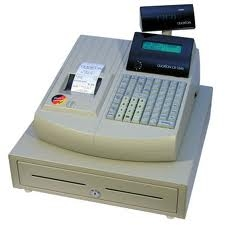# Ten cashiers

Ten cashiers are open at Tesco. Customers wait an average of 15 minutes. How many other cashiers have to open to reduce the waiting time by 4 minutes?Did you find an error or inaccuracy? Feel free to write us. Thank you!Tips to related online calculators
Looking for help with calculating arithmetic mean?
Looking for calculator of harmonic mean?
Looking for a statistical calculator?
Check out our ratio calculator.
Do you have a linear equation or system of equations and looking for its solution? Or do you have a quadratic equation?
Do you want to round the number?
Do you want to convert time units like minutes to seconds?

#### You need to know the following knowledge to solve this word math problem:

We encourage you to watch this tutorial video on this math problem: